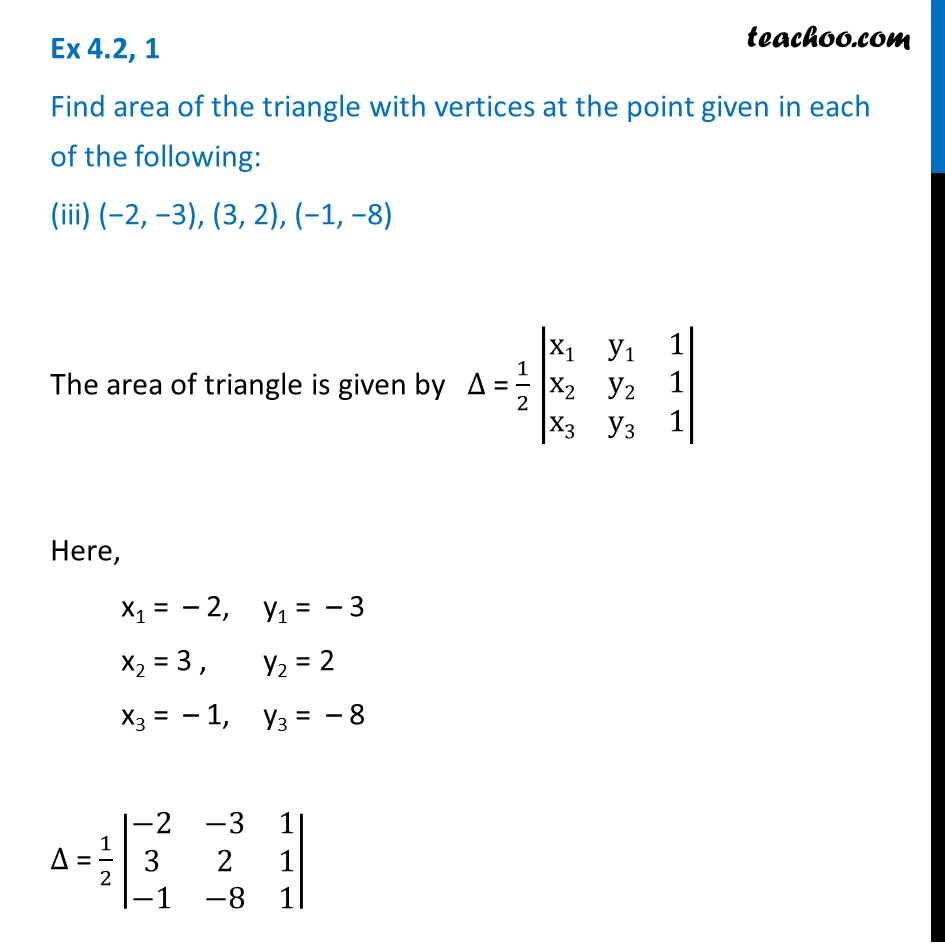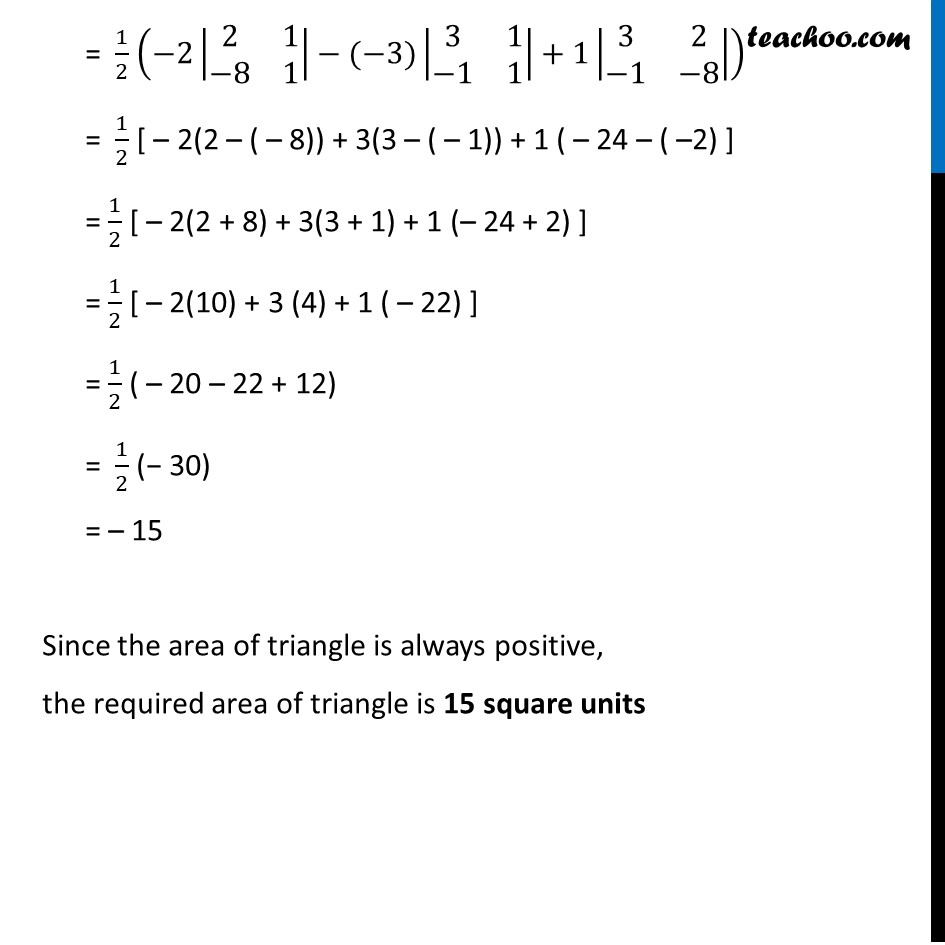Ex 4.2

Chapter 4 Class 12 Determinants
Serial order wiseLearn in your speed, with individual attention - Teachoo Maths 1-on-1 Class

### Transcript

Ex 4.2, 1 Find area of the triangle with vertices at the point given in each of the following: (iii) (−2, −3), (3, 2), (−1, −8) The area of triangle is given by ∆ = 1/2 |■8(x1&y1&1@x2&y2&1@x3&y3&1)| Here, x1 = – 2, y1 = – 3 x2 = 3 , y2 = 2 x3 = – 1, y3 = – 8 ∆ = 1/2 |■8(−2&−3&1@3&2&1@−1&−8&1)| = 1/2 (−2|■8(2&1@−8&1)|−(−3)|■8(3&1@−1&1)|+1|■8(3&2@−1&−8)|) = 1/2 [ – 2(2 – ( – 8)) + 3(3 – ( – 1)) + 1 ( – 24 – ( –2) ] = 1/2 [ – 2(2 + 8) + 3(3 + 1) + 1 (– 24 + 2) ] = 1/2 [ – 2(10) + 3 (4) + 1 ( – 22) ] = 1/2 ( – 20 – 22 + 12) = 1/2 (− 30) = – 15 Since the area of triangle is always positive, the required area of triangle is 15 square units